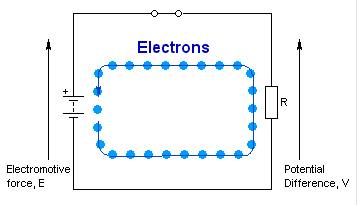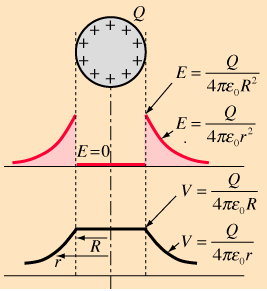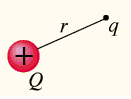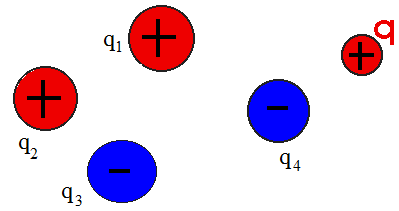×#### Thank you for registering.

One of our academic counsellors will contact you within 1 working day.

Click to Chat

1800-1023-196

+91-120-4616500

CART 0

• 0

MY CART (5)

Use Coupon: CART20 and get 20% off on all online Study Material

ITEM
DETAILS
MRP
DISCOUNT
FINAL PRICE
Total Price: Rs.

There are no items in this cart.
Continue Shopping• Complete Physics Course - Class 11
• OFFERED PRICE: Rs. 2,968
• View Details

Electric Potential

Table of Content

Potential at point due to Several Charges

Potential due to charged Spherical Shell

Potential due to a uniformly charged non-conducting Sphere

Potential at any point due to an Electric Dipole

Electric Potential Energy of a System

Conceptual Question

Related ResourcesThe electric potential is a scalar quantity which is generally denoted by φ, φE or V. It is also termed as electric field potential or the electrostatic potential.

Electric potential, at any point, is defined as the negative line integral of electric field from infinity to that point along any path.

Electric potential is a scalar quantity.

Potential difference, between any two points, in an electric field is negative line integral of electric field between them along any curve joining them together.

In simply words, potential difference between any two points, in an electric field is defined ads the work done in taking a unit positive charge from one point to the other against the electric field.

WAB = q [VA-VB]

So, V = [VA-VB] = W/q

Units:- volt (S.I), stat-volt (C.G.S)

Dimension:- [V] = [ML2T-3A-1]

Relation between volt and stat-volt:- 1 volt = (1/300) stat-volt

The electric potential at any point is equal to the quotient of the electric potential energy of the particle and the charge of the particle at a particular point. The potential energy is measured in joules while the charge is measured in coulombs. The potential may be calculated in either a static or a dynamic electric field and as described above its unit is joules per coulomb i.e. JC-1 or volts V.

The potential energy for a positive charge has inverse relationship with electric field. The energy increases when it moves against an electric field and falls when it moves with the electric field. For a negative charge, the situation is quite opposite.

Potential at point due to Several Charges

V = (1/4π ε0) [q1/r1 + q2/r2 + q3/r3]

= V1+V2+ V3+….

Potential due to charged Spherical ShellTo obtain the electric potential of a charged spherical shell, first we have to fin d out the electric filed inside and outside a thin uniformly charged spherical shell of radius R and charge Q.

The charge distribution is spherical, then E is radial, and its magnitude depends only on the distance r from the center of the sphere. Therefore the Gaussian surface is the spherical surface that has the same center as the spherical shell of charge, of radius r < R inside and r> R outside.

The electric flux inside the shell will be,

ΦE = ∫ E(r). dS = E(r) ∫ dS = 4πr2 E(r)

As we know, the charge inside this surface is zero, thus,

ΣQi = 0.

In accordance to Gauss’s law,

ΦE(inside) = 0

That is, E(inside) = 0

So the potential remains constant at the value it reaches at the surface.

Thus, Vinside = kQ/R = (1/4π ε0) (Q/R)

The flux outside is : ΦE= ∫ E(r). dS = E(r) ∫ dS = 4πr2 E(r)

The charge inside this surface is q/εo.

In accordance to Gauss’s law,

ΦE(outside) = 4πr2 E(r) = Q/εo

Solving for E(r), we get,

E(outside) = Q/4πεo (1/r2)

Thus elecrtric potential will be,

Voutside=Er

=[Q/4πεo (1/r2)]r

=Q/4πεor

At the surface of the charged spherical shell, r = R.

Therefore, Vsurface = Q/4πεoR

Refer this simulation for electric potential and work

We know,W=qV. I use this animation to show electric potential and contrast it with potential energy. It shows a micro sized tractor pushing charges towards a charged block. Using a "work" meter it shows the energy it takes to move the charge. Have the student conclude the relationship between the work and the number of charges. Then relate this to "W=qV."

Potential due to a uniformly charged non-conducting Sphere

(a) Outside, Vout = (1/4π ε0) (q/r)

(b) Inside, Vin = (1/4π ε0) [q(3R2-r2)/2R3]

(c) On the surface, Vsurface = (1/4π ε0) (q/R)

(d) In center, Vcenter = (3/2) [(1/4π ε0) (q/R)] = 3/2 [Vsurface]

Potential at any point due to an Electric Dipole

V (r,θ) = qa cosθ/4πε0r2 = p cosθ/4πε0r2

(a) Point lying on the axial line:- V = p/4πε0r2

(b) Point situated on equatorial lines:- V = 0

Refer this video to know more about electric potential

Electric Potential Energy of a System(a) A system of two charges

The total work done in making an assembly of charges by bringing them from infinity to their respective places is known as the potential energy of the system.

Potential energy is said to be positive if the charges are brought together against the forces of repulsion between them and negative if the charges move under the effect of attractive forces.

Thus, the electric potential energy for point charges q1 and q2 separated by a distance r12, will be,

U = q1q2/ 4π∈0r12

(b) A system of three charges[q1, q2 and q3]

Let three charges q1,q2 and q3 constituting the system. These three charges from three pairs q1q2,q1q3 and q2q3 respectively.

The net potential energy of the system is the sum total of potential energies required to assemble these three pairs separately.

Thus the net potential energy of the system will be,

U = 1/ 4π∈0 (q1q2/r12 + q1q3/r13 + q2q3/r23)

(c) Potential energy at point due to several chargesIf there are ‘N’ charges constituting the configuration, the total number of possible pairs for computing potential energy of the system are,

12,13,14,...., 1N, 23, 24, …..,2N, 34, 35,....,3N and so on .

A general expression for the potential energy of ‘N’ charges can be written as,

Here,

.

The factor ½ appears in the expression due to the reason that the combinations qjqk and qkqj are to be counted as one.

Conceptual Question

During a thunderstorm, a lightning strike places a huge static charge on your car. Why don’t you notice this charge as long as you remain inside the car?

The accumulated charge is all on the outside of the conducting car, so it will only affect you if you step outside and offer it a conducting path to the ground.

Why:

Since the car body is an electrical conductor and its charges are in equilibrium, the car has a uniform voltage and there is no electric field inside the car. Outside the car, however, there is a substantial electric field. If your body provides a conducting path to the ground, that electric field will push charges through you and you will experience a shock. Similarly, if electric power lines ever fall on your car during a storm, stay inside the car to avoid a potentially lethal shock.Electric potential is a analogous to height in mechanics and temperature in heat.

Electric potential is a scalar quantity.

It is the negative line integral of electric field between infinity and the given point.

Electric potential at any point varies inversely as the distance of the point from source charge.

Electric potential at any point due to a positive charge is positive while that due to a negative charge is negative.

Electric Potential Energy is not   the same as Electrical Potential.

Electrical Potential can also be described by the terms, potential difference, voltage, potential drop, potential rise, electromotive force, and EMF.  These terms may differ slightly in meaning depending on the situation.

The variable we use for potential difference is V and the unit for potential difference is also V (volts).  Don’t let that confuse you when you see V = 1.5V

The electron volt is not a smaller unit of the volt, it’s a smaller unit of the Joule.

Problem (JEE Main):

A point charge q is palced at a distance of r from the center of an uncharged conducting sphere of radius R(<r). The potential at any point on the sphere is,

(a) zero                      (b) (1/4π ε0) (q/r)

(c) (1/4π ε0) (qR/r2)    (d)  (1/4π ε0) (qr2/R)

Solution:

Since, potential V is same for all points of the sphere. Therefore, we can calculate its value at the center of the sphere.

Thus, V = (1/4π ε0) (q/r) + V ‘

V ‘=potential at center due to induced charges = 0

Because net induced charge will be zero.

Therefore, V = (1/4π ε0) (q/r)

From the above observation, we conclude that, option (b) is correct.

Problem:

A particle of charge q1 = +6.0 µC  is located on the x-axis at the point x1 = 5.1 cm. A second particle of charge q2 = -5.0 µC is placed on the x-axis at x2 = -3.4 cm. What is the absolute electric potential at the origin (x = 0)? How much work must we perform in order to slowly move a charge of q3 = -7.0  µC from infinity to the origin, whilst keeping the other two charges fixed?

Solution:

The absolute electric potential at the origin due to the first charge is

V1 = keq1/x1

= (8.988109) (610-6)/(5.110-2)

= 1.06106V

Likewise, the absolute electric potential at the origin due to the second charge is

V2= keq2/x2

= (8.988109) (-510-6)/(3.410-2)

= -1.32106V

The net potential V at the origin is simply the algebraic sum of the potentials due to each charge taken in isolation. Thus,

V = V1+V2 = -2.64105 V

The work W which we must perform in order to slowly moving a charge q3 from infinity to the origin is simply the product of the charge and the potential difference V between the end and beginning points. Thus,

W = q3V

= (-710-6) (-2.64105) = 1.85 J

Problem :

Question: Suppose that three point charges, qa, qb, and qc, are arranged at the vertices of a right-angled triangle, as shown in the diagram. What is the absolute electric potential of the third charge if qa = -6.0 µC qb = +4.0 µC qc = +2.0 µC, a = 4.0 m, and b = 3.0 m? Suppose that the third charge, which is initially at rest, is repelled to infinity by the combined electric field of the other two charges, which are held fixed. What is the final kinetic energy of the third charge?Solution:

The absolute electric potential of the third charge due to the presence of the first charge is

Va = keqa/c

= (8.988109) (-610-6)/( √ 42+32)

= -1.08104 V,

where use has been made of the Pythagorean theorem. Likewise, the absolute electric potential of the third charge due to the presence of the second charge is

Vb= keqb/b

= (8.988109) (-410-6)/(3)

= 1.20104 V,

The net absolute potential of the third charge Vc is simply the algebraic sum of the potentials due to the other two charges taken in isolation. Thus,

Vc = Va+Vb = 1.20103V.

The change in electric potential energy of the third charge as it moves from its initial position to infinity is the product of the third charge, qc , and the difference in electric potential (-Vc) between infinity and the initial position. It follows that

?P = – qcVc

= – (210-6) (1.2103)

= – 2.4010-3J

This decrease in the potential energy of the charge is offset by a corresponding increase ?K = – ?P in its kinetic energy. Since the initial kinetic energy of the third charge is zero (because it is initially at rest), the final kinetic energy is simply

K = ?K = – ?P =  – 2.4010-3JQuestion 1

When we rub a glass rod with a silk cloth then

(a) glass rod acquires negative charge while silk acquires positive charge

(b) glass rod acquires positive charge while silk acquires negative charges

(c) both glass rod and silk acquire negative charge

(d) both glass rod and silk acquire positive charge

Question 2

If free space between the plates of a capacitor is replaced by a dielectric

(a) The potential difference remains constant capacitance and energy stored increases

(b) The potential difference remains constant capacitance decreases and energy increases

(c)The potential difference decreases but both capacitance and energy increase

(d) both potential difference and capacitance decrease but energy increases

Question 3

Potential difference is the work done in moving unit positive charge form one point to another

(a) in the direction of electric intensity

(b) against electric intensity

(c)  in any direction

(d) in the direction of electric flux

Question 4

Equipotential planes are

(a) parallel to one another

(b) non parallel to one another

(c) intersecting

(d) circular

Question 5

A hollow metallic sphere of 8cm diameter is charged with 4 x 10 8C. The potential on its surface will be

(a) 900 volts          (b) 9000 volts

(c) 90 volts             (d) zeroQ.1
Q.2
Q.3
Q.4
Q.5

b

c

b

a

b

Related Resources

You might like to refer Energy Stored in a Capacitor.

For getting an idea of the type of questions asked, refer the  Previous Year Question Papers.

To read more, Buy study material of Electrostatics comprising study notes, revision notes, video lectures, previous year solved questions etc. Also browse for more study materials on Physics here.### Course Features

• 101 Video Lectures
• Revision Notes
• Previous Year Papers
• Mind Map
• Study Planner
• NCERT Solutions
• Discussion Forum
• Test paper with Video Solution